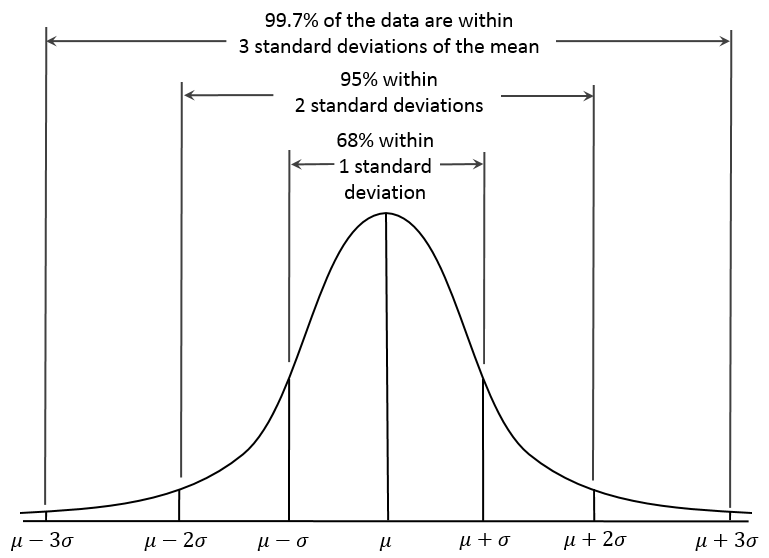# 700 Followers Problem!

Algebra Level 5Expanding $(1+0.2)^{1000}$ by the Binomial theorem gives

${1000 \choose 0}(0.2)^0+{1000 \choose 1}(0.2)^1+{1000 \choose 2}(0.2)^2+\cdots+{1000 \choose 1000}(0.2)^{1000}$ $= A_0 + A_1 + A_2 + \cdots + A_{1000}$

Where $A_k = {1000 \choose k}(0.2)^k$ for $k = 0,1,2,\ldots,1000$.

For which $k_{}^{}$ is $A_k^{}$ the largest?

I Thank all my followers!

###### Image Credit: Wikimedia Empirical Rule by Dan Kernler
×

Problem Loading...

Note Loading...

Set Loading...